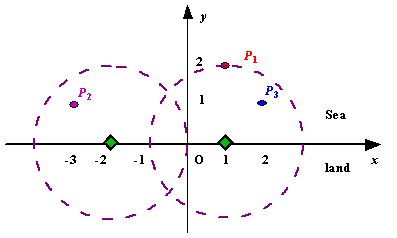贪心 同时被 2 个专栏收录12 篇文章 0 订阅

 Time Limit: 1000MS Memory Limit: 10000K Total Submissions: 45922 Accepted: 10252

Description

Assume the coasting is an infinite straight line. Land is in one side of coasting, sea in the other. Each small island is a point locating in the sea side. And any radar installation, locating on the coasting, can only cover d distance, so an island in the sea can be covered by a radius installation, if the distance between them is at most d.

We use Cartesian coordinate system, defining the coasting is the x-axis. The sea side is above x-axis, and the land side below. Given the position of each island in the sea, and given the distance of the coverage of the radar installation, your task is to write a program to find the minimal number of radar installations to cover all the islands. Note that the position of an island is represented by its x-y coordinates.Figure A Sample Input of Radar Installations

Input

The input consists of several test cases. The first line of each case contains two integers n (1<=n<=1000) and d, where n is the number of islands in the sea and d is the distance of coverage of the radar installation. This is followed by n lines each containing two integers representing the coordinate of the position of each island. Then a blank line follows to separate the cases.

The input is terminated by a line containing pair of zeros

Output

For each test case output one line consisting of the test case number followed by the minimal number of radar installations needed. "-1" installation means no solution for that case.

Sample Input

3 2
1 2
-3 1
2 1

1 2
0 2

0 0


Sample Output

Case 1: 2
Case 2: 1


Source

# include<stdio.h>
# include<string.h>
# include<math.h>
# include<iostream>
# include<algorithm>
using namespace std;

typedef struct Point
{
double left;
double right;
}p;

int cmp(p x,p y)
{
return x.left < y.left;
}

int main()
{
int i,n,k,r,x,y,flag,sum;
double t;
p point;
k = 0;
while(~scanf("%d%d",&n,&r) && n!=0 || r!=0)
{
flag = 1;
for(i=0;i<n;i++)
{
scanf("%d%d",&x,&y);
if(abs(y)>r)
flag = 0;

point[i].left = x*1.0 - sqrt(r*r*1.0 - y*y*1.0);
point[i].right = x*1.0 + sqrt(r*r*1.0 - y*y*1.0);
}

sort(point,point+n,cmp);

sum = 1;
t = point.right;
for(i=1;i<n;i++)
{
if(point[i].right<t)
{
t = point[i].right;
}
else if(point[i].left>t)
{
t = point[i].right;
sum++;
}
}

printf("Case %d: ",++k);

if(flag==0)
printf("-1\n");
else
printf("%d\n",sum);

}

return 0;
}

07-2741012-133457
04-16141
03-1087
01-05447
08-30468
05-14207
07-1983
07-29258
08-20262
04-06129
02-2899
10-1041
10-13212
10-11662
10-12905点击重新获取扫码支付余额充值